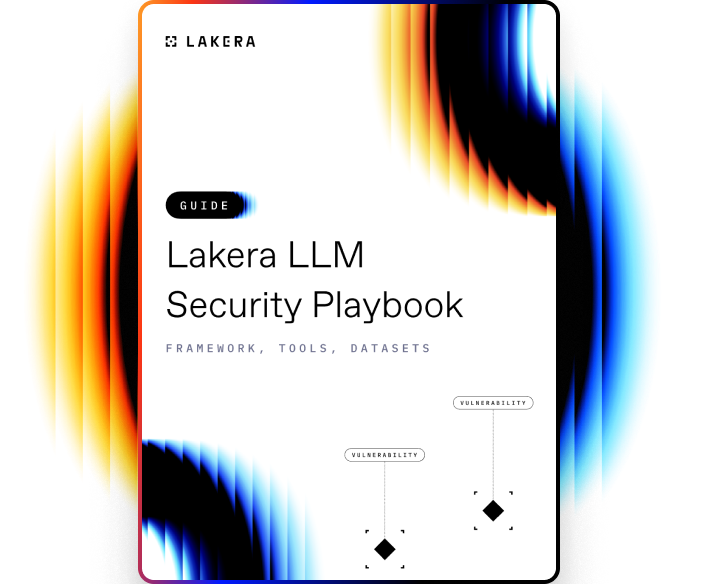VOCABULARY

# Local Interpretable Model-Agnostic Explanations (LIME)

LIME (Local Interpretable Model-Agnostic Explanations) is a method that aims at explaining the predictions of any machine learning classifier in a way that humans can understand. It is a model interpretation tool that breaks down the decision-making process of complex models and helps to understand how the model is taking its decisions.

## How LIME works

LIME constructs a local linear model around each prediction made by a machine learning model to explain why the model made such a prediction. LIME does this by generating a new dataset consisting of perturbed samples, and then trains a linear model on the new dataset to approximate the predictions of the original model.

It starts by choosing an instance that needs explanation, then alters this instance to create a permutation of altered instances. The models are subsequently trained on this new, permuted data set. The key here is that simpler models, which are easier to interpret, are trained on a simplified dataset that is representative of the prediction task at the local level.

The explanations produced by LIME are interpretable, as they are represented as a list of weighted features, where the weights indicate the contribution of each feature to the prediction. This means that the explanation can easily be visualized and understood by humans, which makes LIME a very useful tool for model interpretation.

By using LIME, we can better understand and trust the model's predictions, uncover potential biases in the model's training data, and find out when the model might be giving a prediction based on irrelevant or incorrect features.Learn how to protect against the most common LLM vulnerabilities

Download this guide to delve into the most common LLM security risks and ways to mitigate them.

Related termsActivate
untouchable mode.